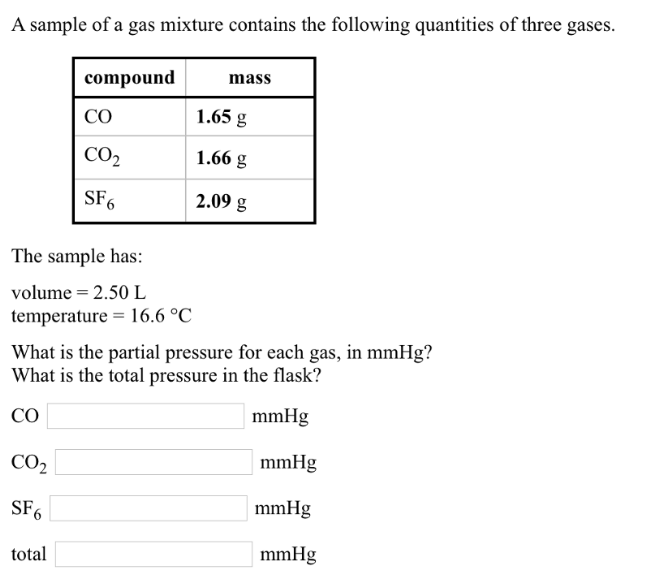# Problem: A sample of a gas mixture contains the following quantities of three gases. The sample has: volume = 2.50 L temperature = 16.6°C. What is the partial pressure for each gas, in mmHg? What is the total pressure in the flask?

###### FREE Expert Solution
79% (210 ratings)###### Problem Details

A sample of a gas mixture contains the following quantities of three gases. The sample has: volume = 2.50 L temperature = 16.6°C. What is the partial pressure for each gas, in mmHg? What is the total pressure in the flask?